# 4  Descriptive Statistics, Distributions, and Graphics

flowchart LR
Types[Types of<br>Measurements] --> Bin[Binary<br><br>Minimum<br>Information] & un[Unordered<br>Categorical<br><br>Low Precision] & ord[Ordered]
ord --> ordd["Ordinal<br>Discrete<br><br>↑Well-Populated<br>Categories→<br>↑Information"] & ordc[Ordinal Continuous]
ordc --> int[Interval Scaled] & ni[Not Interval<br>Scaled]
un & ord --> hist[Histogram]
un --> dot[Dot Chart]
ord --> ecdf[Empirical Cumulative<br>Distribution Function]
ordc --> box[Box Plot<br>Scatterplot]
int --> dens[Nonparametric<br>Density Function]
ordc --> q[Quantiles, IQR]
int --> msd["Mean, SD<br>Gini's mean Δ"]


Relationships Between Y and Continuous X

flowchart LR
mpy[Mean or Proportion<br>of Y vs. X] --> nps[Nonparametric<br>Smoother]
mpy --> ms[Moving<br>General<br>Statistic]
oth["Quantiles of Y<br>Gini's mean Δ<br>SD<br>Kaplan-Meier<br>vs. X"] --> ms
icx[Inherently<br>Categorical X] --> Tab[Table]
mx[Multiple X] --> mod[Model-Based] --> mcharts[Partial<br>Effect Plots<br><br>Effect Differences<br>or Ratios<br><br>Nomograms]
mod --> hm[Color Image<br>Plot to Show<br>Interaction<br>Surface]


## 4.1 Distributions

The distribution of a random variable $$X$$ is a profile of its variability and other tendencies. Depending on the type of $$X$$, a distribution is characterized by the following.

ABD 1.4
• Binary variable: the probability of “yes” or “present” (for a population) or the proportion of same (for a sample).
• $$k$$-Category categorical (polytomous, multinomial) variable: the probability that a randomly chosen person in the population will be from category $$i, i=1,\ldots,k$$. For a sample, use $$k$$ proportions or percents.
• Continuous variable: any of the following 4 sets of statistics
• probability density: value of $$x$$ is on the $$x$$-axis, and the relative likelihood of observing a value “close” to $$x$$ is on the $$y$$-axis. For a sample this yields a histogram.
• cumulative probability distribution: the $$y$$-axis contains the probability of observing $$X\leq x$$. This is a function that is always rising or staying flat, never decreasing. For a sample it corresponds to a cumulative histogram1
• all of the quantiles or percentiles of $$X$$
• all of the moments of $$X$$ (mean, variance, skewness, kurtosis, …)
• If the distribution is characterized by one of the above four sets of numbers, the other three sets can be derived from this set
• Ordinal Random Variables
• Because there many be heavy ties, quantiles may not be good summary statistics
• The mean may be useful if the spacings have reasonable quantitative meaning
• The mean is especially useful for summarizing ordinal variables that are counts
• When the number of categories is small, simple proportions may be helpful
• With a higher number of categories, exceedance probabilities or the empirical cumulative distribution function are very useful
• Knowing the distribution we can make intelligent guesses about future observations from the same series, although unless the distribution really consists of a single point there is a lot of uncertainty in predicting an individual new patient’s response. It is less difficult to predict the average response of a group of patients once the distribution is known.
• At the least, a distribution tells you what proportion of patients you would expect to see whose measurement falls in a given interval.
• 1 But this empirical cumulative distribution function can be drawn with no grouping of the data, unlike an ordinary histogram.

• ### 4.1.1 Continuous Distributions

Code
x <- seq(-3, 35, length=150)
# spar makes base graphics look better
spar <- function(bot=0, top=0, ...)
par(mar=c(3+bot, 2.75+top, .5, .5), mgp=c(2, .475, 0), ...)
spar(mfrow=c(1,2)); xl <- expression(x)
plot(x, dt(x, 4, 6), type='l', xlab=xl, ylab='')
plot(x, pt(x, 4, 6), type='l', xlab=xl, ylab='')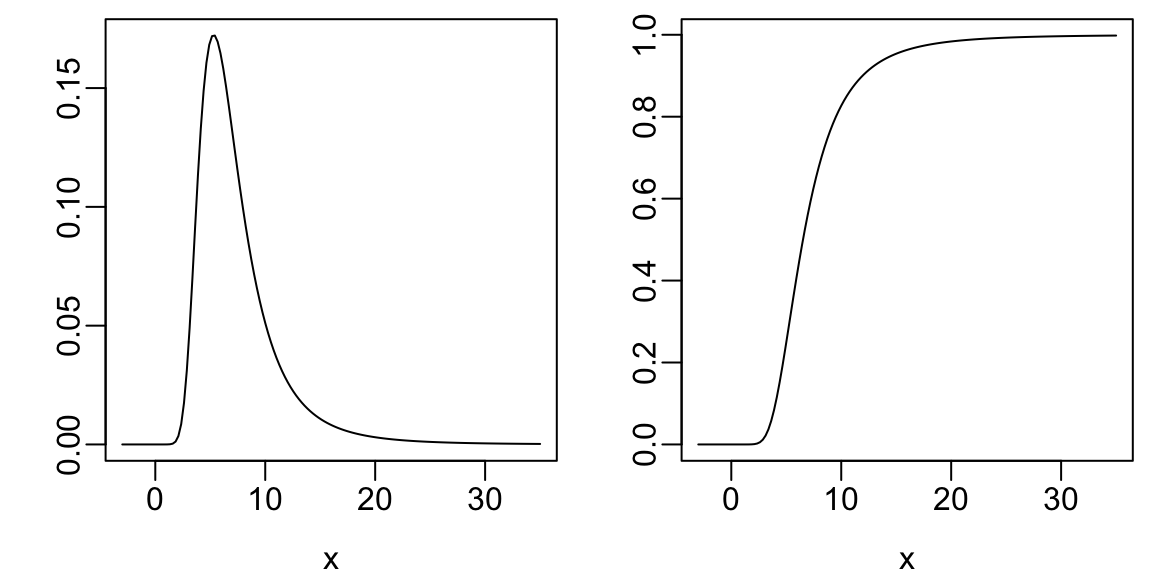Figure 4.1: Example probability density (a) and cumulative probability distribution (b) for a positively skewed random variable (skewed to the right)
Code
spar()
set.seed(1); x <- rnorm(1000)
hist(x, nclass=40, prob=TRUE, col=gray(.9), xlab=xl, ylab='', main='')
x <- seq(-4, 4, length=150)
lines(x, dnorm(x, 0, 1), col='blue', lwd=2)Figure 4.2: Example of a continuous distribution that is symmetric: the Gaussian (normal) distribution with mean 0 and variance 1, along with a histogram from a sample of size 1000 from this distribution
Code
spar()
set.seed(2)
x <- c(rnorm(500, mean=0, sd=1), rnorm(500, mean=6, sd=3))
hist(x, nclass=40, prob=TRUE, col=gray(.9), xlab=xl, ylab='', main='')
lines(density(x), col='blue', lwd=2)
abline(v=c(0, 6), col='red')Figure 4.3: Example of a bimodal distribution from sampling from a mixture of normal distributions with different means and variances and estimating the underlying density function. Vertical red lines indicate true population means of the two component populations. Such a distribution can occur naturally or by failing to condition on a binary characteristic such as sex.

### 4.1.2 Ordinal Variables

• Continuous ratio-scaled variables are ordinal
• Not all ordinal variables are continuous or ratio-scaled
• Best to analyze ordinal response variables using nonparametric tests or ordinal regression
• Heavy ties may be present
• Often better to treat count data as ordinal rather than to assume a distribution such as Poisson or negative binomial that is designed for counts
• Poisson or negative binomial do not handle extreme clumping at zero
• Example ordinal variables are below
Code
spar()
x <- 0:14
y <- c(.8, .04, .03, .02, rep(.01, 11))
plot(x, y, xlab=xl, ylab='', type='n')
segments(x, 0, x, y)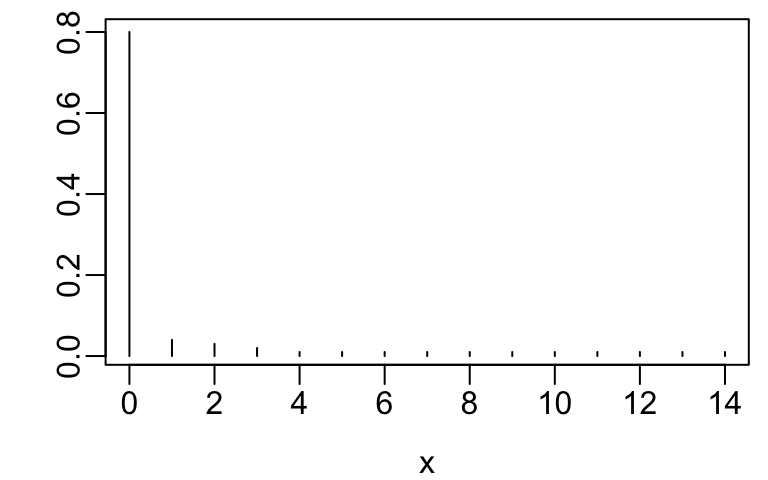Figure 4.4: Distribution of number of days in the hospital in the year following diagnosis
Code
spar()
x <- 1:10
y <- c(.1, .13, .18, .19, 0, 0, .14, .12, .08, .06)
plot(x, y, xlab=xl, ylab='', type='n')
segments(x, 0, x, y)Figure 4.5: Distribution of a functional status score that does not have points in the middle

The getHdata function in the Hmisc package Harrell (2020a) finds, downloads, and load()s datasets from hbiostat.org/data.

Code
spar()
require(Hmisc)
getHdata(nhgh)   # NHANES dataset    Fig. (* @fig-descript-ordc*):
yl <- label(esopH$orophar) ggplot(esopH, aes(x=conv, y=orophar)) + geom_point(pch='.') + xlab(xl) + ylab(yl) + geom_abline(intercept = 0, slope = 1)Figure 4.12: Scatterplot of one measurement mode against another With large sample sizes there are many collisions of data points and hexagonal binning is an effective substitute for the raw data scatterplot. The number of points represented by one hexagonal symbol is stratified into 15 groups of approximately equal numbers of points. Code ggplot(esopH, aes(x=conv, y=orophar)) + stat_binhex(aes(fill=Hmisc::cut2(..count.., g=15)), bins=40) + xlab(xl) + ylab(yl) + guides(fill=guide_legend(title='Frequency'))Figure 4.13: Hexagonal binning replacing scatterplot for large $$n$$ Use the Hmisc ggfreqScatter function to bin the points and represent frequencies of overlapping points with color and transparency levels. Code with(esopH, ggfreqScatter(conv, orophar, bins=50, g=20) + geom_abline(intercept=0, slope=1))Figure 4.14: Binned points (2500 total bins) with frequency counts shown as color and transparency level #### Distributions ABD 1.4 • histogram showing relative frequencies • requires arbitrary binning of data • not optimal for comparing multiple distributions • cumulative distribution function: proportion of values $$\leq x$$ vs. $$x$$ (Figure 4.15) Can read all quantiles directly off graph. Code getHdata(pbc) pbcr <- subset(pbc, drug != 'not randomized') spar(bot=1) Ecdf(pbcr[,c('bili','albumin','protime','sgot')], group=pbcr$drug, col=1:2,
label.curves=list(keys='lines'))Figure 4.15: Empirical cumulative distributions of baseline variables stratified by treatment in a randomized controlled trial. $$m$$ is the number of missing values.
• Box plots shows quartiles plus the mean. They are a good way to compare many groups as shown below.
Note that this approach is very ineffective in handling continuous baseline variables
Code
require(lattice)
getHdata(diabetes)
wst <- cut2(diabetes\$waist, g=2)
levels(wst) <- paste('Waist', levels(wst))
bwplot(cut2(age,g=4) ~ glyhb | wst*gender, data=diabetes,
panel=panel.bpplot, xlab='Glycosylated Hemoglobin', ylab='Age Quartile')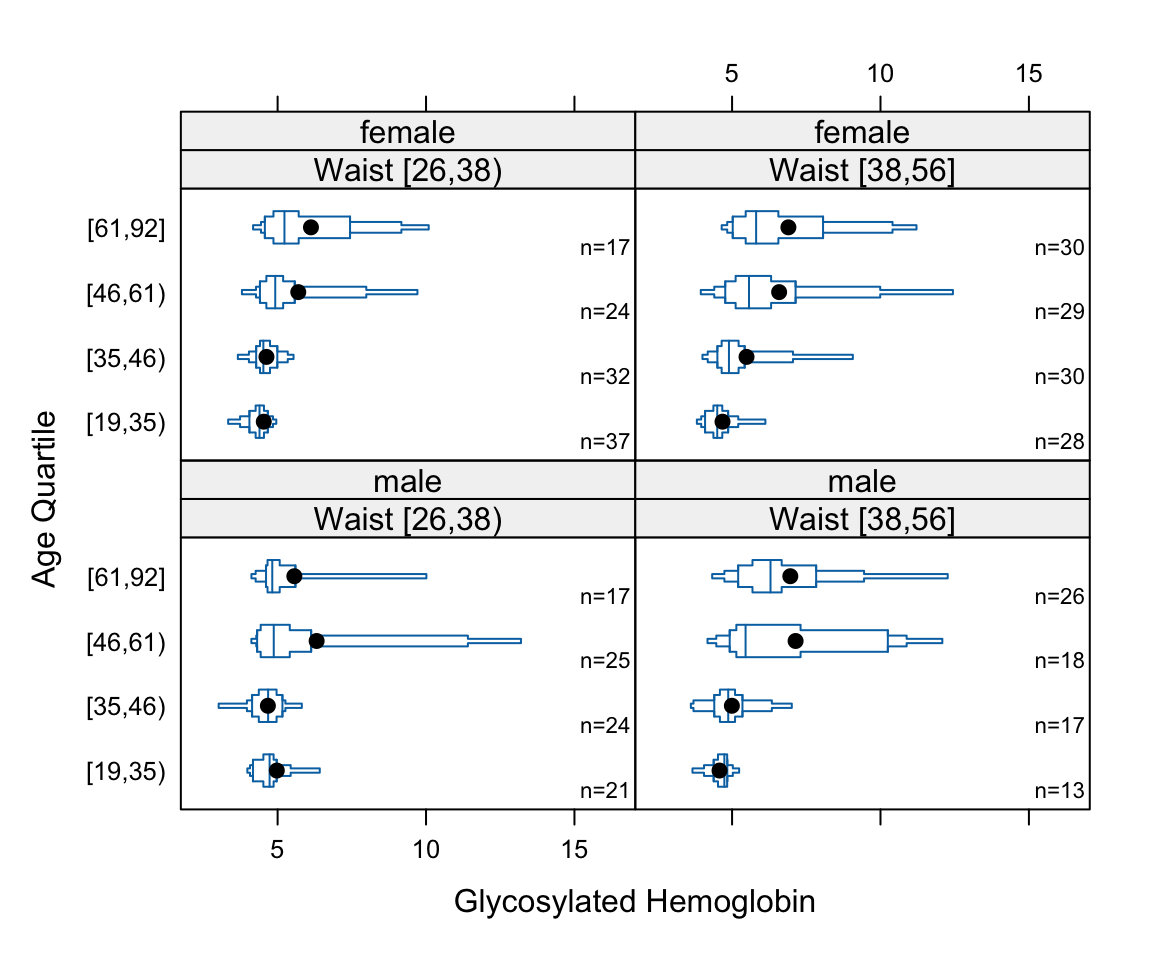Figure 4.16: Box plots for glycohemoglobin stratified by quartiles of age (vertical), two-tiles of waist circumference (horizontal), and sex (vertical)

Regular box plots have a high ink:information ratio. Using extended box plots we can show more quantiles. The following schematic shows how to interpret them.

Code
bpplt()

Here are extended box plots stratified by a single factor.

Code
getHdata(support)
s <- summaryM(crea ~ dzgroup, data=support)
# Note: if the variables on the left side of the formula contained a mixture of
# categorical and continuous variables there would have been two parts to to
# results of plot(s), one named Continuous and one named Categorical
plot(s)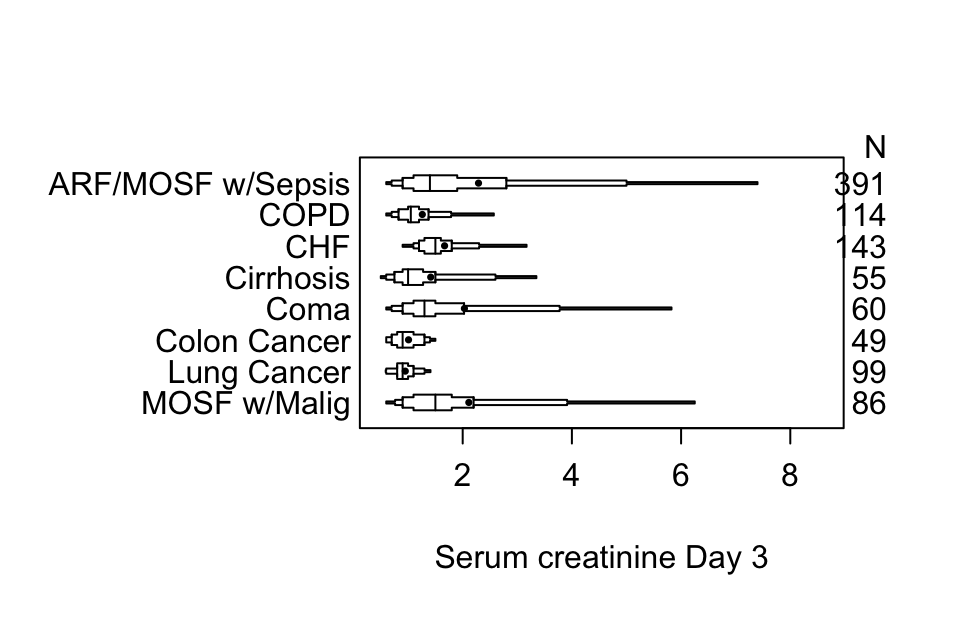Figure 4.18: Extended plots showing the distribution of serum creatinine stratified by major diagnosis. Asymmetry of distributions can be seen by both disagreement between $$Q_{3}-Q_{2}$$ and $$Q_{2}-Q_{1}$$ and by disagreement between $$Q_{2}$$ and $$\bar{x}$$.

Using plotly graphics we can make an interactive extended box plot whose elements are self-explanatory; hover the mouse over graphical elements to see their definitions and data values.

Code
options(grType='plotly')   # set to use interactive graphics output for
# certain Hmisc and rms package functions
plot(s)                    # default is grType='base'
Code
options(grType='base')

Box plots (extended or not) are inadequate for displaying bimodality. Violin plots show the entire distribution well if the variable being summarized is fairly continuous.

Even better are spike histograms, when not also showing longitudinal trends.Figure 4.20: One-half violin plots for longitudinal data, stratified by treatment. Density estimates for groups with insufficient sample sizes are faded. Density plots are back-to-back for treatment A and B. Points are treatment medians. When the black vertical line does not touch the two medians, the medians are significantly different at the $$\alpha=0.05$$ level. Graphic was produced by the R greport package.

#### Relationships

• When response variable is continuous and descriptor (stratification) variables are categorical, multi-panel dot charts, box plots, multiple cumulative distributions, etc., are useful.
• Categorization of continuous independent variable X is highly problematic and misleading
• Two continuous variables: scatterplot
• Trends when X is continuous
• nonparametric smoother such as loess for trends in means or proportions
• more general: moving statistics (generalizations of moving averages)
• can estimate quantiles, mean, variability of Y vs. X
• can also allow for censoring, e.g., moving 1y and 2y cumulative incidence using the Kaplan-Meier estimator
• general R function movStats
• Moving statistics method
• does not categorize X into non-overlapping intervals
• creates small intervals of X, compute the statistic of interest on Y
• move to the next interval, overlapping significantly with the last window
• link the Y statistic computed in the X window to the mean X within the interval
• after getting Y statistics for all overlapping X intervals, smooth these X-Y pairs using the “super-smoother” or loess
• windows are formed based on interval sample sizes, not based on absolute coordinates; allows for highly skewed X-distributions (window sizes vary, in X units)
• Example: moving 2-year cumulative incidence of disease
• first interval is from the lowest X to the 25th lowest X
• compute Y statistic for those observations: one minus the Kaplan-Meier estimate of 2y event-free probability
• attach to mean X in the 25 lowest Xs
• move up 5 observations, forming a new interval from the 6th lowest X to the 30th lowest X
• as we get away from the outer part of the X distribution the windows will be $$\pm 15$$ observations on either side of the target X for which the Y property is being estimated
• when finished apply a large amount of smoothing to the X-Y pairs

As an example let’s estimate the upper quartile of glycohemoglobin (HbA$$_{\mathrm{1c}}$$) and the variability in HbA$$_{\mathrm{1c}}$$, both as a function of age. Our measure of variability will be Gini’s mean difference. Use an NHANES dataset. The raw age distribution is shown in the first panel. Instead of using the default window sample size of $$\pm 15$$ observations use 30.

Code
getRs('movStats.r')   # Load function from Github
require(data.table)
getHdata(nhgh)
setDT(nhgh)
d <- nhgh[, .(age, gh)]
stats <- function(y)
list('Moving Q3'   = quantile(y, 3/4),
'Moving Gmd'  = GiniMd(y),
N             = length(y))
u <- movStats(gh ~ age, stat=stats, msmooth='both',
eps=30,  # +/- 30 observation window
melt=TRUE, data=d, pr='margin')
Window Sample Sizes
N Mean Min Max xinc
6795 60.8 40 61 33
Code
g <- ggplot(u, aes(x=age, y=gh)) + geom_line(aes(color=Type)) +
facet_wrap(~ Statistic, scale='free_y') +
xlim(10, 80) + xlab('Age') + ylab(expression(HbA["1c"]))
g <- addggLayers(g, d, value='age', facet=list(Statistic='Gmd'))
facet=list(Statistic='Gmd'))Figure 4.21: Moving upper quartile and Gini’s mean difference of HbA$$_{\mathrm 1c}$$ as a function of age for NHANES, with and without smoothing. An extended box plot and spike histogram is included in the first facet (Gmd for Gini’s mean difference) to show the age distribution. The extended box plot is supplemented by 0.01 and 0.99 quantiles (outer dots).

Younger subjects tend to differ from each other in HbA$$_{\mathrm{1c}}$$ by 0.5 on the average while typical older subjects differ by almost 1.0. Note the large spike in the histogram at age 80. NHANES truncated ages at 80 as a supposed safeguard against subject re-identification.

Re-draw the last graph but just for the smoothed estimates for $$Q_3$$, and include a spike histogram.

Code
u <- u[Type == 'Moving-smoothed' & Statistic == 'Q3',]
g <- ggplot(u, aes(x=age, y=gh)) + geom_line() +
xlab('Age') + ylab(expression(paste(HbA["1c"]~~~Q))) + ylim(5.4, 6.4)
addggLayers(g, d, value='age', type='spike', pos='bottom')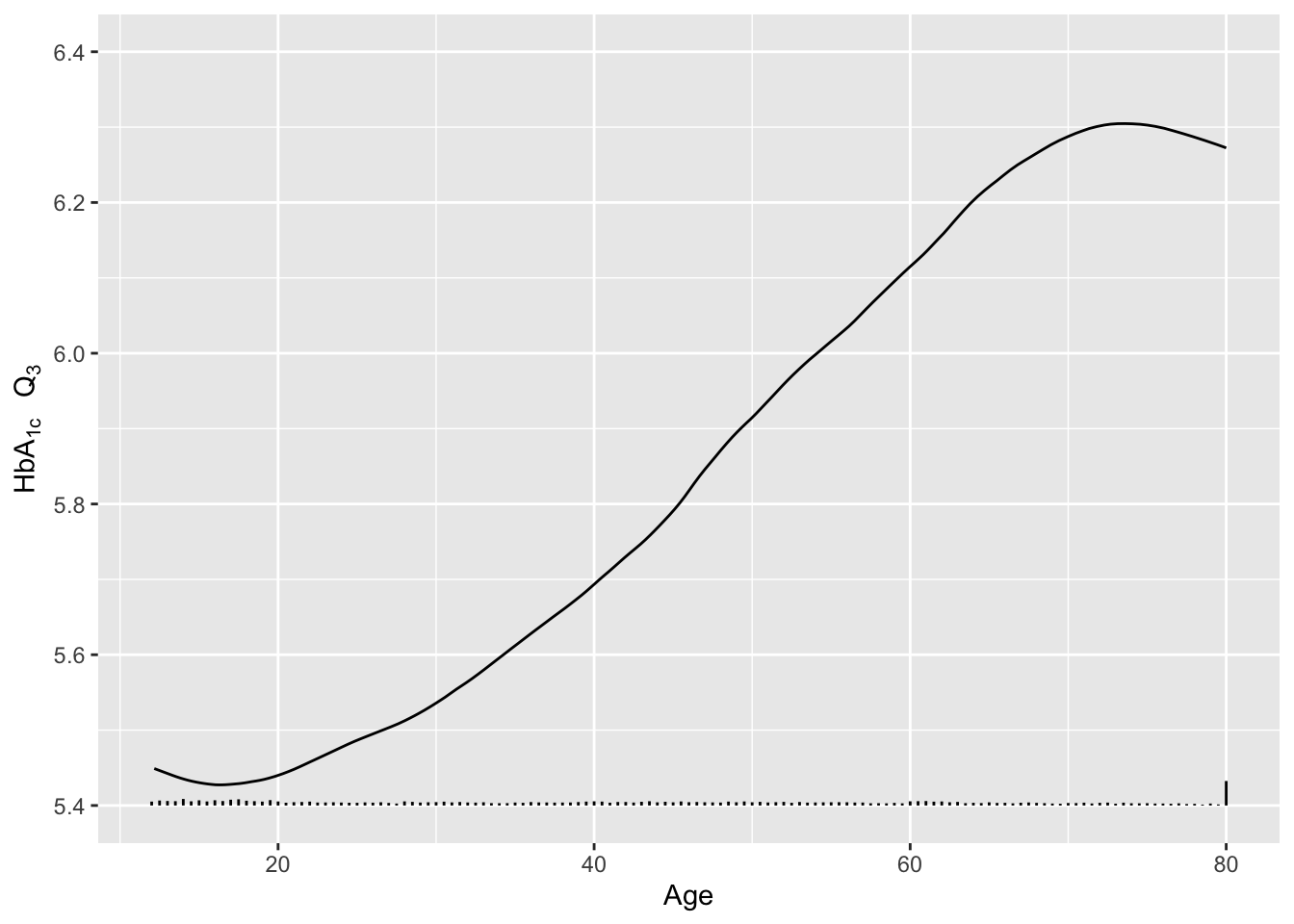Figure 4.22: Moving $$Q_3$$ as a function of age with a spike histogram depicting the age distribution.

### 4.3.4 Graphs for Summarizing Results of Studies

• Dot charts with optional error bars (for confidence limits) can display any summary statistic (proportion, mean, median, mean difference, etc.)
• It is not well known that the confidence interval for a difference in two means cannot be derived from individual confidence limits.4
Show individual confidence limits as well as actual CLs for the difference.
• 4 In addition, it is not necessary for two confidence intervals to be separated for the difference in means to be significantly different from zero.

• Code
attach(diabetes)
set.seed(1)
male   <- smean.cl.boot(glyhb[gender=='male'],   reps=TRUE)
female <- smean.cl.boot(glyhb[gender=='female'], reps=TRUE)
dif <- c(mean=male['Mean']-female['Mean'],
quantile(attr(male, 'reps')-attr(female,'reps'), c(.025,.975)))
plot(0,0,xlab='Glycated Hemoglobin',ylab='',
xlim=c(5,6.5),ylim=c(0,4), axes=F)
axis(1, at=seq(5, 6.5, by=0.25))
axis(2, at=c(1,2,3.5), labels=c('Female','Male','Difference'),
points(c(male,female), 2:1)
segments(female, 1, female, 1)
segments(male, 2,   male, 2)
offset <- mean(c(male,female)) - dif
points(dif + offset, 3.5)
segments(dif+offset, 3.5, dif+offset, 3.5)
at <- c(-.5,-.25,0,.25,.5,.75,1)
axis(3, at=at+offset, label=format(at))
segments(offset, 3, offset, 4.25, col=gray(.85))
abline(h=c(2 + 3.5)/2, col=gray(.85))Figure 4.23: Means and nonparametric bootstrap 0.95 confidence limits for HbA$$_{\mathrm 1c}$$ for males and females, and confidence limits for males - females. Lower and upper $$x$$-axis scales have same spacings but different centers. Confidence intervals for differences are generally wider than those for the individual constituent variables.
• For showing relationship between two continuous variables, a trend line or regression model fit, with confidence bands

#### Bar Plots with Error Bars

• “Dynamite” Plots
• Height of bar indicates mean, lines represent standard error
• High ink:information ratio
• Hide the raw data, assume symmetric confidence intervals
• Replace with
• Dot plot (smaller sample sizes)
• Box plot (larger sample size)
Code
getHdata(FEV); set.seed(13)
FEV <- subset(FEV, runif(nrow(FEV)) < 1/8)   # 1/8 sample
require(ggplot2)
s <- with(FEV, summarize(fev, llist(sex, smoke), smean.cl.normal))
ggplot(s, aes(x=smoke, y=fev, fill=sex)) +
geom_bar(position=position_dodge(), stat="identity") +
geom_errorbar(aes(ymin=Lower, ymax=Upper),
width=.1,
position=position_dodge(.9))

See biostat.app.vumc.org/DynamitePlots for a list of the many problems caused by dynamite plots, plus some solutions.

Instead of the limited information shown in the bar chart, show the raw data along with box plots. Modify default box plots to replace whiskers with the interval between 0.1 and 0.9 quantiles.

Code
require(ggplot2)
stats <- function(x) {
z <- quantile(x, probs=c(.1, .25, .5, .75, .9))
names(z) <- c('ymin', 'lower', 'middle', 'upper', 'ymax')
if(length(x) < 10) z[c(1,5)] <- NA
z
}
ggplot(FEV, aes(x=sex, y=fev)) +
stat_summary(fun.data=stats, geom='boxplot', aes(width=.75), shape=5,
position='dodge', col='lightblue') +
geom_dotplot(binaxis='y', stackdir='center', position='dodge', alpha=.4) +
stat_summary(fun.y=mean, geom='point', shape=5, size=4, color='blue') +
facet_grid(~ smoke) +
xlab('') + ylab(expression(FEV)) + coord_flip()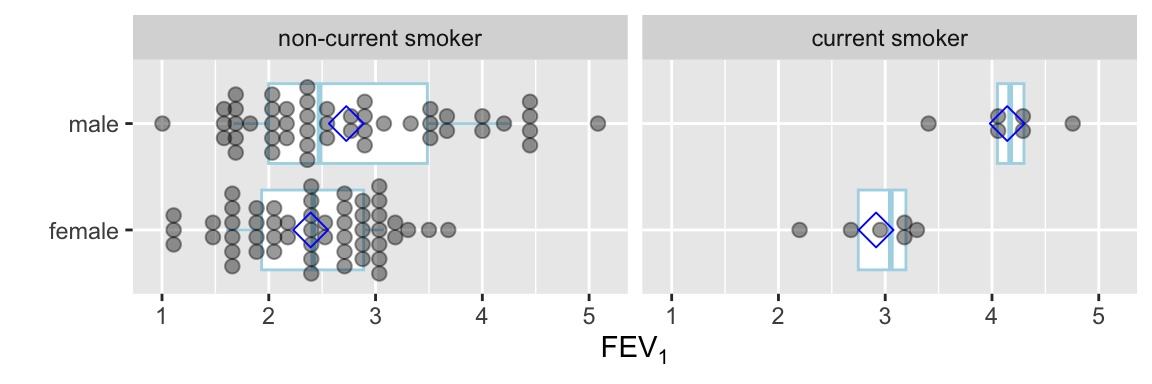Figure 4.25: Jittered raw data and box plots. Middle vertical lines indicate medians and diamonds indicate means. Horizontal lines indicate 0.1 to 0.9 quantiles when $$n\geq 10$$. The ink:information ratio for this plot is far better than a dynamite plot.

Use a violin plot to show the distribution density estimate (and its mirror image) instead of a box plot.

Code
ggplot(FEV, aes(x=sex, y=fev)) +
geom_violin(width=.6, col='lightblue') +
geom_dotplot(binaxis='y', stackdir='center', position='dodge', alpha=.4) +
stat_summary(fun.y=median, geom='point', color='blue', shape='+', size=12) +
facet_grid(~ smoke) +
xlab('') + ylab(expression(FEV)) + coord_flip()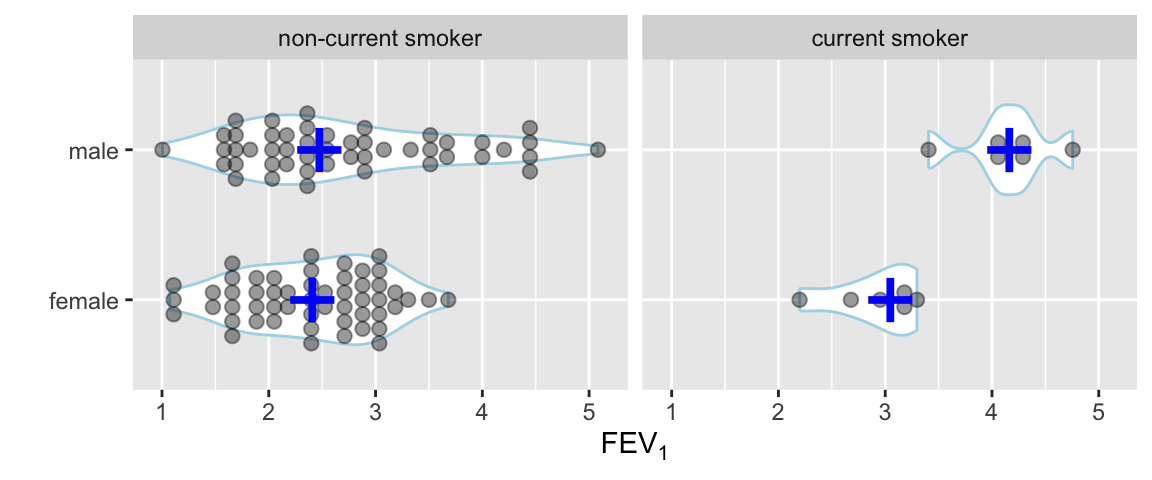Figure 4.26: Jittered raw data and violin plots with median indicated by blue +

#### Semi-Interactive Graphics Examples

These examples are all found in hbiostat.org/talks/rmedicine19.html.

Other examples: descriptions of BBR course participants: hbiostat.org/bbr/registrants.html.

### 4.3.5 Graphs for Describing Statistical Model Fits

Several types of graphics are useful. All but the first are implemented in the R rms package Harrell (2020b).

• Single-axis nomograms: Used to transform values (akin to using a slide rule to compute square roots) or to get predicted values when the model contains a single predictor
• Partial effect plots: Show the effect on $$Y$$ of varying one predictor at a time, holding the other predictors to medians or modes, and include confidence bands. This is the best approach for showing shapes of effects of continuous predictors.
• Effect charts: Show the difference in means, odds ratio, hazard ratio, fold change, etc., varying each predictor and holding others to medians or modes5. For continuous variables that do not operate linearly, this kind of display is not very satisfactory because of its strong dependence on the settings over which the predictor is set. By default inter-quartile-range effects are used.
• Nomograms: Shows the entire model if the number of interactions is limited. Nomograms show strengths and shapes of relationships, are very effective for continuous predictors, and allow computation of predicted values (although without confidence limits).
• 5 It does not matter what the other variables are set to if they do not interact with the variable being varied.

• For an example of a single-axis nomogram, consider a binary logistic model of a single continuous predictor variable X from which we obtain the predicted probability of the event of interest.

The intercept $$\alpha$$ and slope $$\beta$$ were computed to match a published model. These would ordinarily be computed from coef(f) where f is the fit object.
Code
par(mar=c(3,.5,1,.5))
beta  <- (qlogis(.999)-qlogis(.001))/177
alpha <- qlogis(.001)-23*beta
plot.new()
par(usr=c(-10,230, -.04, 1.04))
par(mgp=c(1.5,.5,0))
axis(1, at=seq(0,220,by=20))
axis(1, at=seq(0,220,by=5), tcl=-.25, labels=FALSE)
title(xlab='X')
p <- c(.001,.01,.05,seq(.1,.9,by=.1),.95,.99,.999)
s <- (qlogis(p)-alpha) / beta
axis(1, at=s, label=as.character(p), col.ticks=gray(.7),
tcl=.5, mgp=c(-3,-1.7,0))
p <- c(seq(.01,.2,by=.01), seq(.8,99,by=.01))
s <- (qlogis(p)-alpha) / beta
axis(1, at=s, labels=FALSE, col.ticks=gray(.7), tcl=.25)
title(xlab='Estimated Risk', mgp=c(-3,-1.7,0))

Here are examples of graphical presentations of a fitted multivariable model using NHANES data to predict glycohemoglobin from age, sex, race/ethnicity, and BMI.. Restricted cubic spline functions with 4 default knots are used to allow age and BMI to act smoothly but nonlinearly. Partial effects plots are in Figure 4.28

Ordinary regression is not an adequate fit for glycohemoglobin; an excellent fit comes from ordinal regression. BMI is not an adequate summary of body size. An ordinary regression model in the $$-1.75$$ power of glycohemoglobin resulted in approximately normal residuals and is used for illustration. The transformation is subtracted from a constant just so that positive regression coefficients indicate that increasing a predictor increases glycohemoglobin. The inverse transformation raises predicted values to the $$\frac{-1}{1.75}$$ power after accounting for the subtraction, and is used to estimate the median glycohemoglobin on the original scale. If residuals have a normal distribution after transforming the dependent variable, the estimated mean and median transformed values are the same. Inverse transforming the estimates provides an estimate of the median on the original scale (but not the mean).
Code
require(rms)
getHdata(nhgh)   # NHANES data
g        <- function(x) 0.09 - x ^ - (1 / 1.75)
ginverse <- function(y) (0.09 - y) ^ -1.75
f <- ols(g(gh) ~ rcs(age, 4) + re + sex + rcs(bmi, 4), data=nhgh)
cat('<br><small>\n')

Code
f

Linear Regression Model

ols(formula = g(gh) ~ rcs(age, 4) + re + sex + rcs(bmi, 4), data = nhgh)

Model Likelihood
Ratio Test
Discrimination
Indexes
Obs 6795 LR χ2 1861.16 R2 0.240
σ 0.0235 d.f. 11 R2adj 0.238
d.f. 6783 Pr(>χ2) 0.0000 g 0.015

Residuals

      Min        1Q    Median        3Q       Max
-0.097361 -0.012083 -0.002201  0.008237  0.168892 
β S.E. t Pr(>|t|)
Intercept  -0.2884  0.0048 -60.45 <0.0001
age   0.0002  0.0001 3.34 0.0008
age'   0.0010  0.0001 7.63 <0.0001
age''  -0.0040  0.0005 -8.33 <0.0001
re=Other Hispanic  -0.0013  0.0011 -1.20 0.2318
re=Non-Hispanic White  -0.0082  0.0008 -10.55 <0.0001
re=Non-Hispanic Black  -0.0013  0.0009 -1.34 0.1797
re=Other Race Including Multi-Racial   0.0006  0.0014 0.47 0.6411
sex=female  -0.0022  0.0006 -3.90 <0.0001
bmi  -0.0006  0.0002 -2.54 0.0111
bmi'   0.0059  0.0009 6.44 <0.0001
bmi''  -0.0161  0.0025 -6.40 <0.0001
Code
print(anova(f), dec.ss=3, dec.ms=3)
Analysis of Variance for g(gh)
d.f. Partial SS MS F P
age 3 0.732 0.244 441.36 <0.0001
Nonlinear 2 0.040 0.020 35.83 <0.0001
re 4 0.096 0.024 43.22 <0.0001
sex 1 0.008 0.008 15.17 <0.0001
bmi 3 0.184 0.061 110.79 <0.0001
Nonlinear 2 0.023 0.011 20.75 <0.0001
TOTAL NONLINEAR 4 0.068 0.017 30.94 <0.0001
REGRESSION 11 1.181 0.107 194.29 <0.0001
ERROR 6783 3.749 0.001
Code
cat('</small>\n')

Code
# Show partial effects of all variables in the model, on the original scale
ggplot(Predict(f, fun=ginverse),
ylab=expression(paste('Predicted Median ', HbA['1c'])))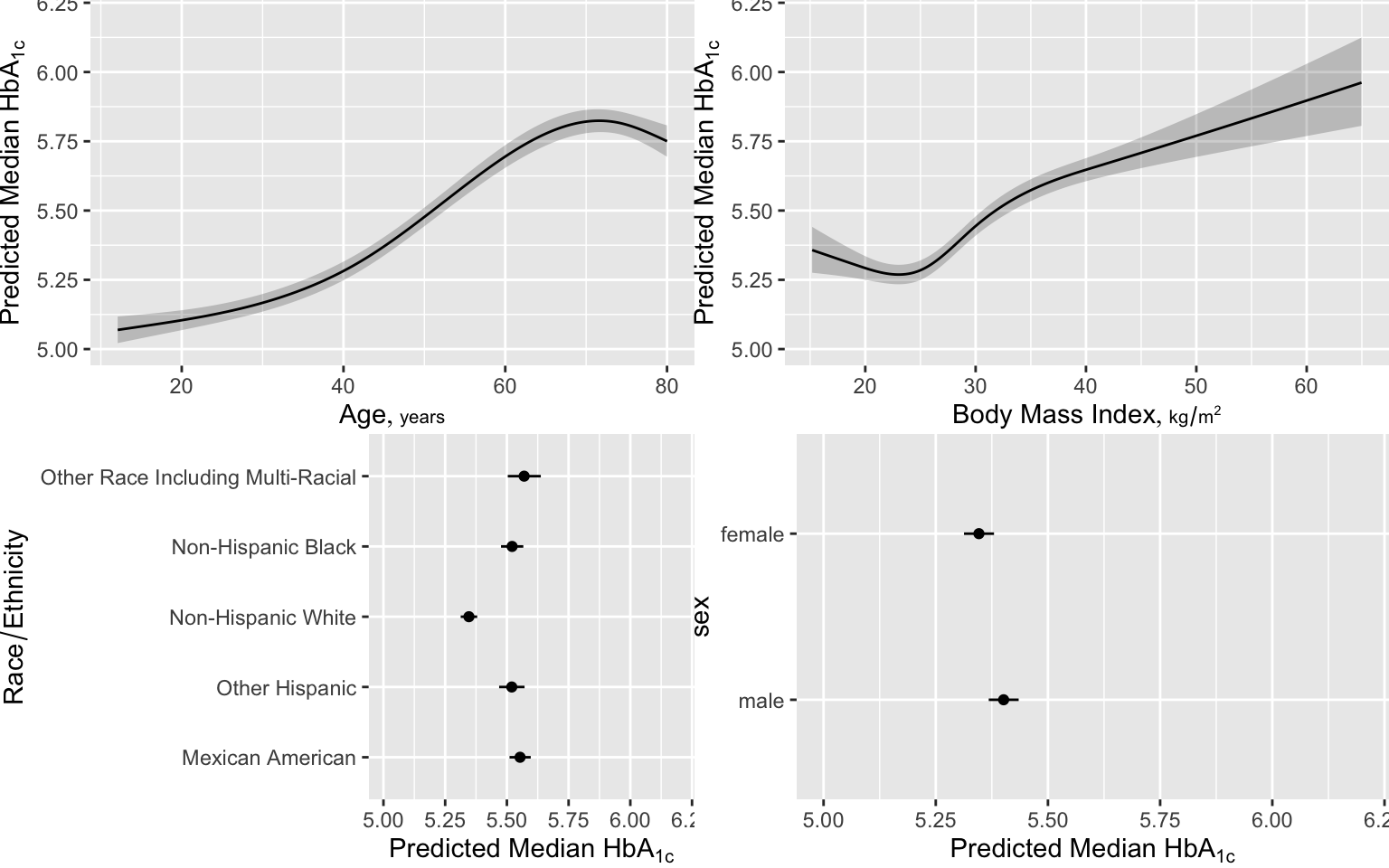Figure 4.28: Partial effects in NHANES HbA$$_{1c}$$ model

An effect chart is in Figure 4.29 and a nomogram is in Figure 4.30. See stats.stackexchange.com/questions/155430/clarifications-regarding-reading-a-nomogram for excellent examples showing how to read such nomograms.

Code
plot(summary(f))Figure 4.29: Partial effects chart on the transformed scale. For age and BMI, effects are inter-quartile-range effects. 0.9, 0.95, and 0.99 confidence limits are shown.
Code
plot(nomogram(f, fun=ginverse, funlabel='Median HbA1c'))Figure 4.30: Nomogram for predicting median HbA$$_{1c}$$. To use the nomogram, use the top Points scale to convert each predictor value to a common scale. Add the points and read this number on the Total Points scale, then read down to the median.

#### Graphing Effect of Two Continuous Variables on $$Y$$

The following examples show the estimated combined effects of two continuous predictors on outcome. The two models included interaction terms, the second example using penalized maximum likelihood estimation with a tensor spline in diastolic $$\times$$ systolic blood pressure.Figure 4.32: Logistic regression estimate of probability of a hemorrhagic stroke for patients in the GUSTO-I trial given $$t$$-PA, using a tensor spline of two restricted cubic splines and penalization (shrinkage). Dark (cold color) regions are low risk, and bright (hot) regions are higher risk.

Figure 4.32 is particularly interesting because the literature had suggested (based on approximately 24 strokes) that pulse pressure was the main cause of hemorrhagic stroke whereas this flexible modeling approach (based on approximately 230 strokes) suggests that mean arterial blood pressure (roughly a $$45^\circ$$ line) is what is most important over a broad range of blood pressures. At the far right one can see that pulse pressure (axis perpendicular to $$45^\circ$$ line) may have an impact although a non-monotonic one.

## 4.4 Tables

What are tables for?

• Lookup of details
• Not for seeing trends
• For displaying a summary of a variable stratified by a truly categorical variable
• Not for summarizing how a variable changes across levels of a continuous independent variable

Since tables are for lookup, they can be complex. With modern media, a better way to think of a table is as a pop-up when viewing elements of a graph.

What to display in a table for different types of response variables:

• Binary variables: Show proportions first; they should be featured because they are normalized for sample size
• Don’t need to show both proportions (e.g., only show proportion of females)
• Proportions are better than percents because of reduced confusion when speaking of percent difference (is it relative or absolute?) and because percentages such as 0.3% are often mistaken for 30% or 3%.
• Make logical choices for independent and dependent variables.
E.g., less useful to show proportion of males for patients who lived vs. those who died than to show proportion of deaths stratified by sex.
• Continuous response variables
• to summarize distributions of raw data: 3 quartiles
recommended format: 35 50 67 or 35/50/67
• summary statistics: mean or median and confidence limits (without assuming normality of data if possible)
• Continuous independent (baseline) variables
• Don’t use tables because these requires arbitrary binning (categorization)
• Use graphs to show smooth relationships
• Show number of missing values
• Confidence intervals: in a comparative study, show confidence intervals for differences, not confidence intervals for individual group summaries
Code
getHdata(stressEcho)
d <- stressEcho
require(data.table)
setDT(d)
d[, hxofMI := factor(hxofMI, 0 : 1, c('No history of MI', 'History of MI'))]
s <- summaryM(bhr + basebp + basedp + pkhr + maxhr + gender~ hxofMI, data=d)
print(s, exclude1=TRUE, npct='both', digits=3, middle.bold=TRUE)
Descriptive Statistics (N=558).
No history of MI
N=404
History of MI
N=154
Basal heart rate
bpm
65 74 85 63 72 84
Basal blood pressure
mmHg
120 134 150 120 130 150
Basal Double Product bhr*basebp
bpm*mmHg
8514 9874 11766 8026 9548 11297
Peak heart rate
mmHg
107 123 136 104 120 132
Maximum heart rate
bpm
107 122 134 102 118 130
gender : female 0.68 273404 0.42 65154
a b c represent the lower quartile a, the median b, and the upper quartile c for continuous variables.